# Review up to the last time

Data analysis for improving POG 2 ~ Analysis with jupyter notebook ~ shows the causal relationship between the horse profile and the prizes won during the POG period. By analyzing, we were able to grasp general trends such as "mares are disadvantageous" and "earlier birth is more advantageous".

# Purpose of this time

Determine the possibility of predicting POG period prizes based on horse profiles by regression analysis.

# Data analysis

## Handling of qualitative data

Let's take a look at the contents of the data to be analyzed again.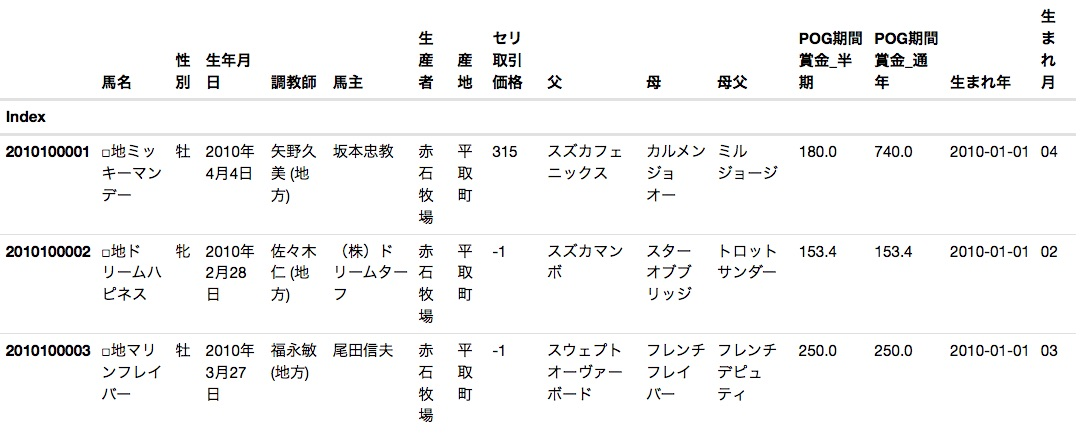Since we want to predict the prize amount based on the profile of each horse, the objective variable is "POG period prize_year-round" and the explanatory variables are "gender", "month of birth", "trainer", "owner", "producer". , "Origin", "Seri transaction price", "Father", "Mother and father" would be appropriate. However, since the "seri transaction price" was not found to have a significant relationship in the previous analysis, it is excluded from the analysis this time.

By the way, among the explanatory variables, the data other than the "seri transaction price" are so-called qualitative data. Therefore, regression analysis cannot be performed as it is.

In such a case, it seems to be a general method * to perform regression analysis after converting qualitative data into dummy variables so that they can be treated as quantitative data.

pandas has a function that converts qualitative data into dummy variables. An example is shown below.

#### `python`

``````
pd.get_dummies(horse_df[u'sex'])[:3]
``````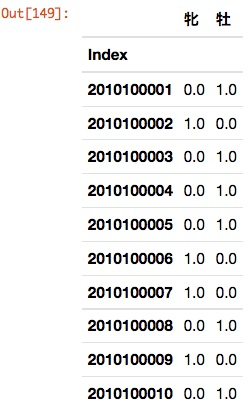## Simple regression analysis

In this analysis, the OLS (least squares method) of the statsmodels module is used. The code used for the analysis is shown below.

#### `python`

``````
#Module import
import pandas as pd
import numpy as np
import matplotlib.pyplot as plt
import seaborn as sns
sns.set(font='Osaka')
import statsmodels.api as sm
import IPython.display as display
%matplotlib inline

#Read analysis source data
#horse_df = horse_df[:50]

#Convert qualitative data to dummy variables
use_col = [
u'sex',
#u'Birth month',
#u'Trainer',
#u'Horse owner',
#u'Producer',
#u'Origin',
#u'father',
#u'Mother father',
]

if len(use_col) == 1:
dum = pd.get_dummies(horse_df[use_col])
else:
dum = pd.get_dummies(horse_df[use_col])

#　X,Definition of y
X_col = dum.columns
y_col = u'POG period prize_Year-round'
tmp_df = pd.concat([dum, horse_df[y_col]], axis=1)
tmp_df = tmp_df.dropna()
tmp_df = tmp_df.applymap(np.int)
X = tmp_df[X_col].ix[:,:]
y = tmp_df[y_col]

#Model generation
model = sm.OLS(y,X)

#result
results = model.fit()
y_predict = results.predict()
plt.plot(y_predict, y, marker='o', ls='None', label='_'.join(use_col))
plt.xlabel(u'Forecast')
plt.ylabel(u'Actual measurement')
plt.legend(loc=0)
plt.title('R^2: %.3f,  F: %.3f' % (results.rsquared, results.fvalue))
plt.savefig('./figure/fig_'+'_'.join(use_col)+'.png')
#display.display(results.summary())
``````

### sex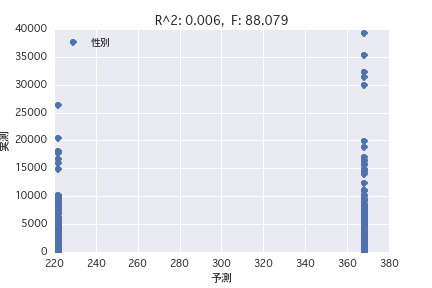### Birth month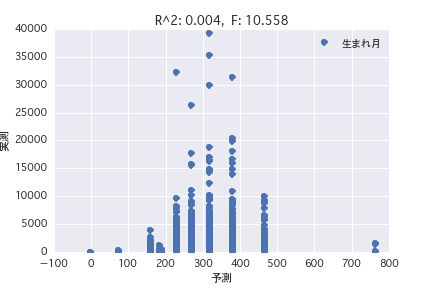### Trainer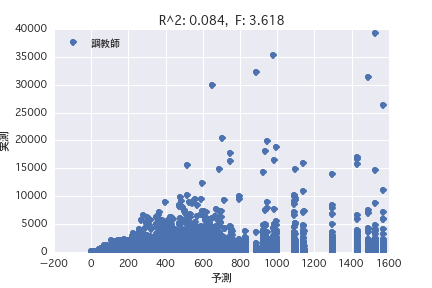### Horse owner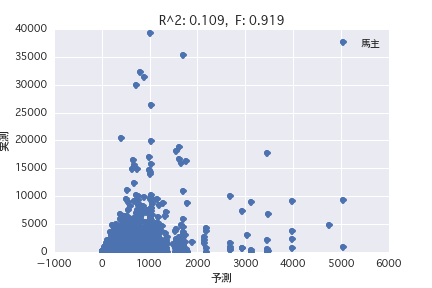### Producer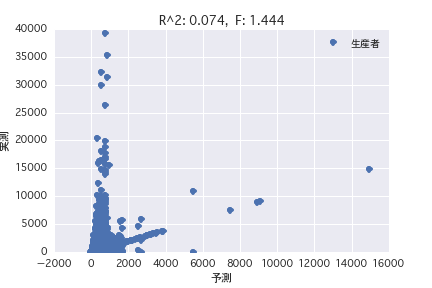### Origin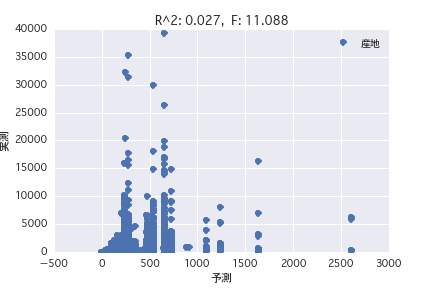### father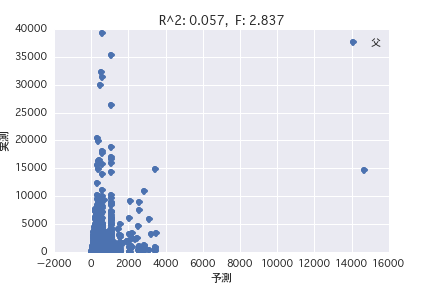### Mother father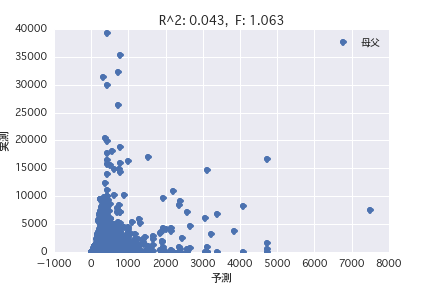## Multiple regression analysis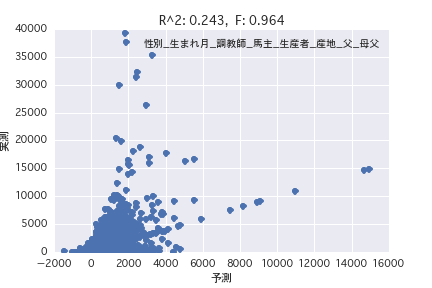# This summary

Regression analysis was performed with the objective variable as "POG period prize_year-round" and the explanatory variable as various horse profiles ("gender", "father", etc.). Both simple regression analysis and multiple regression analysis have a small R ^ 2, and it was found that it is difficult to predict the prize amount from the horse profile.

# from now on

Discriminant analysis (identification of unwinned, average open horses, first-class horses) Analysis focusing on pedigree